## August 28, 2011

### What if PageRank didn’t exist?

Filed under: data analysis,education,mathematics — Peter Saveliev @ 10:30 am

Gotcha! You thought this is about SEO. No, I meant “exist” in the mathematical sense…

When an entity or a concept in mathematics is introduced via a description instead of a direct computation, there is a possibility that this entity doesn’t even exist. This happens when there is nothing that satisfies the description. For example, “define x as a number that satisfies equation x+1=x” produces nothing.

Similarly, when something is introduced via an iterative process instead of a direct computation, does it always make sense? It’s possible that no matter how many iterations you run, you don’t get anything in particular (“divergence”). For example, “start with 1, then add 1/2, then add 1/3, 1/4, etc” leads to unlimited growth with no end.

So, what does this have to do with PageRank?

Let’s review the math behind it. Suppose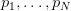$p_1,\ldots ,p_N$ are the$N$ pages/nodes in the graph (of the web). Suppose also that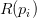$R(p_i)$ is the PageRank of node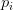$p_i$ and together they form a vector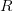$R$. Then the ranking satisfy the “balance equation”:$R(p_i) = d \sum_{p_j \in S(p_i)} \frac{R (p_j)}{L(p_j)} + \frac{1-d}{N},$
where
*$S(p_i)$ is the set of nodes that (inbound) link to$p_i$,
*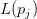$L(p_j)$ is the number of (outbound) links from node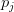$p_j$.

Skipping some algebra here, we discover that the PageRank vector$R$ is found as:$R = d(1-d) \left( \frac{1}{d}I-M \right) ^{-1}R_0,$
where the matrix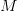$M$ is$M_{ij} = \begin{cases} 1 /L(p_j) & \mbox{if }p_j \in S(p_i),\ \\ 0 & \mbox{if }p_j \notin S(p_i), \end{cases}$
and$R_0(p_i) = \frac{1}{N},i=1,2,\ldots ,N,$ is the initial distribution of rankings.

Problem solved! But before we start celebrating we have to make sure that the inverse matrix in the formula exists!

The Perron–Frobenius theorem ensures that this is the case — but the matrix has to be “irreducible”.

Matrix$M$ is irreducible if and only if the graph with an edge from node$i$ to node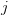$j$ when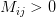$M_{ij} > 0$ is ”strongly connected”, i.e., there is a path from each node to every other node.

But we all know that there are always pages with no inbound links! The proof fails.

So, we have no reason to believe that a PageRank distribution exists for the given state of the web. This means that, no matter how you compute$R$, what comes out will be garbage.

Well, some people aren’t worried about this. Others worry so much that they try to fix it, in ridiculous ways. A common approach, for example, is to add “missing” links. Don’t get me started on that. If you introduce made-up parameters or try to make data fit your model, that’s bad math!

Are there other mathematical problems with PageRank? Still to come.